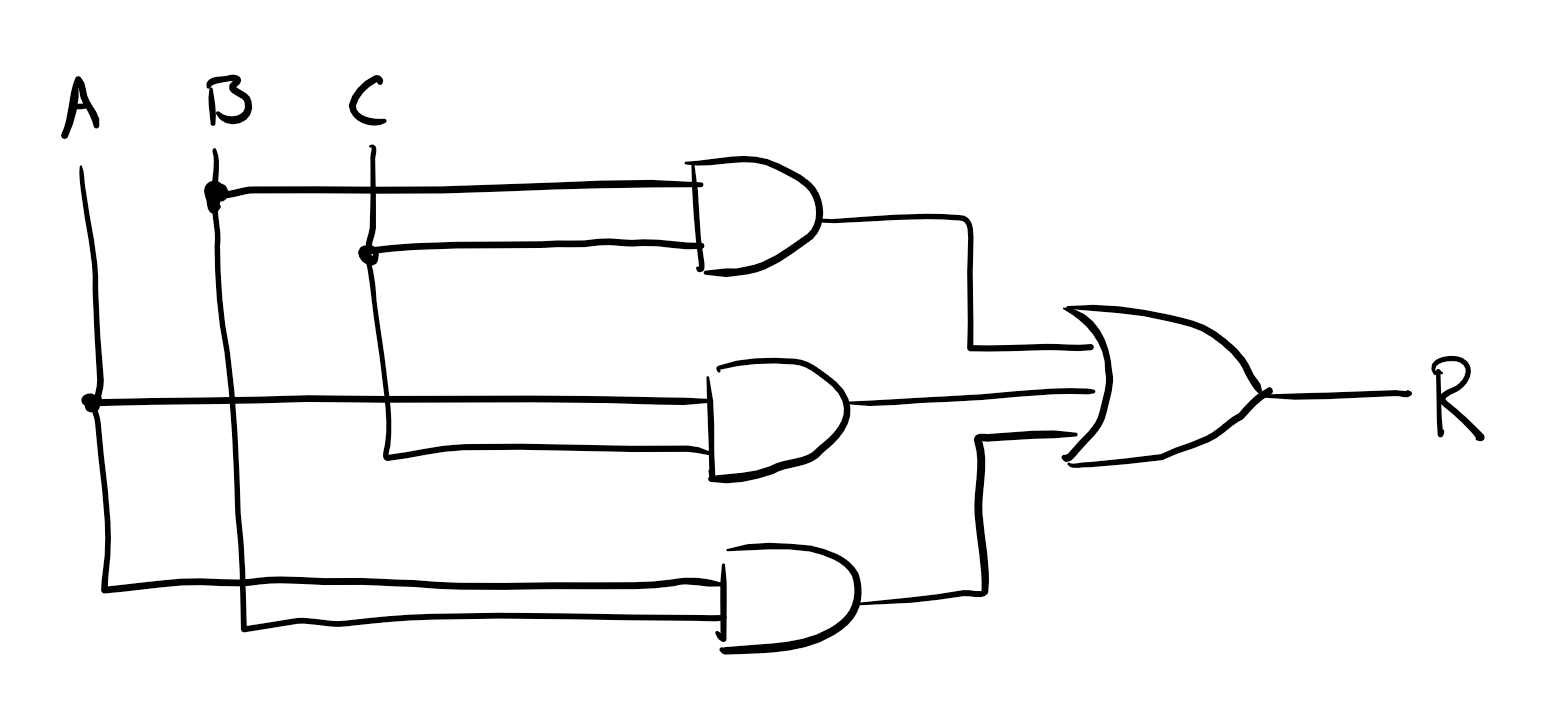Name: Box: Date:

## HW1 solution

(5 points) Write the 8-bit 2's complement form of -25.

11100111

(5 points) Write the hexadecimal form of 1337.

0x539

(10 points) Below is a truth table that implements 3vote. It has inputs A, B, C and output R. Design a digital logic circuit that implements the truth table. You may only use multi-input AND, OR, and NOT gates.

A B C R
0 0 0 0
0 0 1 0
0 1 0 0
0 1 1 1
1 0 0 0
1 0 1 1
1 1 0 1
1 1 1 1

Use sum-of-products to construct the equation:

$$R = \overline{A}BC + A\overline{B}C + AB\overline{C} + ABC$$

This can be simplified to

$$R = BC + AC + AB$$

The solution for the simplified equation uses three 2-input AND gates. The first has B,C, the second A,C, the third A,B. Each AND result is connected to a 3-input OR gate. This OR produces the final output R.(15 points) Write a module in Verilog or a program in C that implements 3vote. You should choose types appropriate for the inputs and outputs.

int threevote(int a, int b, int c) {
int r = (b&&c) || (a&&c) || (a&&b);
return r;
}

or

module threevote(
input a,
input b,
input c,
output r);

assign r = b&c | a&c | a&b;

endmodule